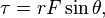# Torque: Applied Forces Pos/Neg?

Hi,

I have a question regarding the forces applied on an object that create the torque. I understand that the resulting torque is pos if the object turns counter clock wise and is neg if it turns clock wise and that you can also use the right hand rule to find the direction of the net torque.

I found the right answer for my homework by making some of the resulting torques negative, but I don't understand why they are negative.

Particularly one such problem where there is a circle. One of the forces is tangent and pointing up toward the E most point of the circle. The answer needs this to be neg, but the right hand rule makes it pos?

Was I suppose to be looking for the difference in the resulting torques? But that doesn't make any sense since the net torque is the sum of the torques found.

Last edited:

PhanthomJay
Homework Helper
Gold Member
Never assign a negative value to a force when determining the direction of the torque. Use right hand rule, clockwise is plus and counterclockwise is negative, or the other way around if you wish.

Conclusion:

When finding the torque, the magnitude is found through the equationbut to find the direction you must use the right hand rule for each individual torque before finding the net torque. The ones in opposite directions will cancel each other out and then you will use the right hand rule again to find the direction of the net torque.

The key is that you must assign opposite signs to torques on ccw and cw motion. It doesn't matter which sign you use although the convention is that ccw is positive and cw is negative.

•Ellispson
CWatters